## Linear-Algebra

 Question 1
Let X be a square matrix. Consider the following two statements on X.
I. X is invertible.
II. Determinant of X is non-zero.
Which one of the following is TRUE?
 A I implies II; II does not imply I. B II implies I; I does not imply II. C I and II are equivalent statements. D I does not imply II; II does not imply I.
Engineering-Mathematics       Linear-Algebra       GATE 2019       Video-Explanation
Question 1 Explanation:
X is invertible means, that X is non-singular matrix.
That means we can also say that determinant of X is non-zero.
 Question 2

Consider the following matrix: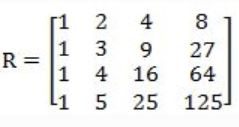The absolute value of the product of Eigen values of R is ______.

 A 12 B 17 C 10 D 8
Engineering-Mathematics       Linear-Algebra       GATE 2019       Video-Explanation
Question 2 Explanation:Question 3The largest eigenvalue of A is ________

 A 3 B 4 C 5 D 6
Engineering-Mathematics       Linear-Algebra       GATE 2018       Video-Explanation
Question 3 Explanation:Correction in Explanation: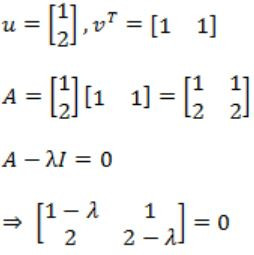⇒ (1 - λ)(2 - λ) - 2 = 0
⇒ λ2 - 3λ=0
λ = 0, 3
So maximum is 3.
 Question 4

Assume that multiplying a matrix G1 of dimension p×q with another matrix G2 of dimension q×r requires pqr scalar multiplications. Computing the product of n matrices G1G2G3…Gn can be done by parenthesizing in different ways. Define GiGi+1 as an explicitly computed pair for a given parenthesization if they are directly multiplied. For example, in the matrix multiplication chain G1G2G3G4G5G6 using parenthesization(G1(G2G3))(G4(G5G6)), G2G3 and G5G6 are the only explicitly computed pairs.

Consider a matrix multiplication chain F1F2F3F4F5, where matrices F1, F2, F3, F4 and F5 are of dimensions 2×25, 25×3, 3×16, 16×1 and 1×1000, respectively. In the parenthesization of F1F2F3F4F5 that minimizes the total number of scalar multiplications, the explicitly computed pairs is/ are

 A F1F2 and F3F4 only B F2F3 only C F3F4 only D F1F2 and F4F5 only
Engineering-Mathematics       Linear-Algebra       GATE 2018       Video-Explanation
Question 4 Explanation:
→ As per above information, the total number of scalar multiplications are 2173.
→ Optimal Parenthesization is:
((F1(F2(F3 F4)))F5)
→ But according to the problem statement we are only considering F3, F4 explicitly computed pairs.
 Question 5

Consider Guwahati (G) and Delhi (D) whose temperatures can be classified as high (H), medium (M) and low (L). Let P(HG) denote the probability that Guwahati has high temperature. Similarly, P(MG) and P(LG) denotes the probability of Guwahati having medium and low temperatures respectively. Similarly, we use P(HD), P(MD) and P(LD) for Delhi.

The following table gives the conditional probabilities for Delhi’s temperature given Guwahati’s temperature.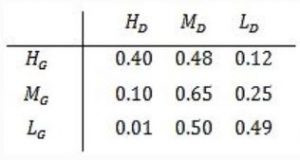Consider the first row in the table above. The first entry denotes that if Guwahati has high temperature (HG) then the probability of Delhi also having a high temperature (HD) is 0.40; i.e., P(HD ∣ HG) = 0.40. Similarly, the next two entries are P(MD ∣ HG) = 0.48 and P(LD ∣ HG) = 0.12. Similarly for the other rows.

If it is known that P(HG) = 0.2, P(MG) = 0.5, and P(LG) = 0.3, then the probability (correct to two decimal places) that Guwahati has high temperature given that Delhi has high temperature is _______ .

 A 0.6 B 0.61 C 0.62 D 0.63
Engineering-Mathematics       Linear-Algebra       GATE 2018       Video-Explanation
Question 5 Explanation: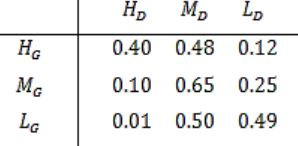The first entry denotes that if Guwahati has high temperature (HG ) then the probability that Delhi also having a high temperature (HD ) is 0.40.
P (HD / HG ) = 0.40
We need to find out the probability that Guwahati has high temperature.
Given that Delhi has high temperature (P(HG / HD )).P (HD / HG ) = P(HG ∩ HD ) / P(HD )
= 0.2×0.4 / 0.2×0.4+0.5×0.1+0.3×0.01
= 0.60
 Question 6
Let N be the set of natural numbers. Consider the following sets,
P: Set of Rational numbers (positive and negative)
Q: Set of functions from {0, 1} to N
R: Set of functions from N to {0, 1}
S: Set of finite subsets of N
Which of the above sets are countable?
 A Q and S only B P and S only C P and R only D P, Q and S only
Engineering-Mathematics       Linear-Algebra       GATE 2018       Video-Explanation
Question 6 Explanation:
Set of rational numbers are countable. It is proved by various methods in literature.
Set of functions from {0,1} to N is countable as it has one to one correspondence to N.
Set of functions from N to {0,1} is uncountable, as it has one to one correspondence to set of real numbers between (0 and 1).
Set of finite subsets of N is countable.
 Question 7
Consider a matrix P whose only eigenvectors are the multiples of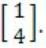.
Consider the following statements.
(I)P does not have an inverse
(II)P has a repeated eigenvalue
(III)P cannot be diagonalized
Which one of the following options is correct?
 A Only I and III are necessarily true B Only II is necessarily true C Only I and II are necessarily true D Only II and III are necessarily true
Engineering-Mathematics       Linear-Algebra       GATE 2018       Video-Explanation
Question 7 Explanation:Though the multiple of a vector represents same vector, and each eigen vector has distinct eigen value, we can conclude that ‘p’ has repeated eigen value.
If the unique eigen value corresponds to an eigen vector e, but the repeated eigen value corresponds to an entire plane, then the matrix can be diagonalized, using ‘e’ together with any two vectors that lie in plane.
But, if all eigen values are repeated, then the matrix cannot be diagonalized unless it is already diagonal.
So (III) holds correct.
A diagonal matrix can have inverse, So (I) is false.
Then (II) and (III) are necessarily True.
 Question 8

Let c1, cn be scalars not all zero. Such that the following expression holds:where ai is column vectors in Rn. Consider the set of linear equations.

Ax = B.

where A = [a1.......an] andThen, Set of equations has

 A a unique solution at x = Jn where Jn denotes a n-dimensional vector of all 1 B no solution C infinitely many solutions D finitely many solutions
Engineering-Mathematics       Linear-Algebra       GATE 2017 [Set-1]       Video-Explanation
Question 8 Explanation:
ai is a column vector
AX = BAs given thatand c1&cn ≠ 0
means c0a0 + c1a1 + ...cnan = 0, represents that a0, a1... an are linearly dependent.
So rank of 'A' = 0, (so if ‘B’ rank is = 0 infinite solution, ‘B’ rank>0 no solution) ⇾(1)
Another condition given here is, 'Σai = b',
so for c1c2...cn = {1,1,...1} set, it is having value 'b',
so there exists a solution if AX = b →(2)
From (1)&(2), we can conclude that AX = B has infinitely many solutions.
 Question 9

Let u and v be two vectors in R2 whose Euclidean norms satisfy ||u||=2||v||. What is the value of α such that w = u + αv bisects the angle between u and v?

 A 2 B 1/2 C 1 D -1/2
Engineering-Mathematics       Linear-Algebra       GATE 2017 [Set-1]       Video-Explanation
Question 9 Explanation:Let u, v be vectors in R2, increasing at a point, with an angle θ.
A vector bisecting the angle should split θ into θ/2, θ/2
Means ‘w’ should have the same angle with u, v and it should be half of the angle between u and v.
Assume that the angle between u, v be 2θ (thus angle between u,w = θ and v,w = θ)
Cosθ = (u∙w)/(∥u∥ ∥w∥) ⇾(1)
Cosθ = (v∙w)/(∥v∥ ∥w∥) ⇾(2)
(1)/(2) ⇒ 1/1 = ((u∙w)/(∥u∥ ∥w∥))/((v∙w)/(∥v∥ ∥w∥)) ⇒ 1 = ((u∙w)/(∥u∥))/((v∙w)/(∥v∥))
⇒ (u∙w)/(v∙w) = (∥u∥)/(∥v∥) which is given that ∥u∥ = 2 ∥v∥
⇒ (u∙w)/(v∙w) = (2∥v∥)/(∥v∥) = 2 ⇾(3)
Given ∥u∥ = 2∥v∥
u∙v = ∥u∥ ∥v∥Cosθ
=2∙∥v∥2 Cosθ
w = u+αv
(u∙w)/(v∙w) = 2
(u∙(u+αv))/(v∙(u+αv)) = 2
(u∙u+α∙u∙v)/(u∙v+α∙v∙v) = 2a∙a = ∥a∥2
4∥v∥2+α∙2∙∥v∥2 Cosθ = 2(2∥v∥2 Cosθ+α∙∥v∥2)
4+2αCosθ = 2(2Cosθ+α)
4+2αCosθ = 4Cosθ+2α ⇒ Cosθ(u-v)+2α-4 = 0
4-2α = Cosθ(4-2α)
(4-2α)(Cosθ-1) = 0
4-2α = 0Question 10
Let A be m×n real valued square symmetric matrix of rank 2 with expression given below.Consider the following statements
(i) One eigenvalue must be in [-5, 5].
(ii) The eigenvalue with the largest magnitude must be strictly greater than 5.
Which of the above statements about eigenvalues of A is/are necessarily CORRECT?
 A Both (I) and (II) B (I) only C (II) only D Neither (I) nor (II)
Engineering-Mathematics       Linear-Algebra       GATE 2017 [Set-1]       Video-Explanation
Question 10 Explanation:
Letbe a real valued, rank = 2 matrix.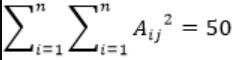a2+b2+c2+d2 = 50
Square values are of order 0, 1, 4, 9, 16, 25, 36, …
So consider (0, 0, 5, 5) then Sum of this square = 0+0+25+25=50
To get rank 2, the 2×2 matrix can beThe eigen values are,
|A-λI| = 0 (The characteristic equation)-λ(-λ)-25 = 0
λ2-25 = 0So, the eigen values are within [-5, 5], Statement I is correct.
The eigen values with largest magnitude must be strictly greater than 5: False.
So, only Statement I is correct.
 Question 11

If the characteristic polynomial of a 3 × 3 matrix M over R (the set of real numbers) is λ3 - 4λ2 + aλ + 30, a ∈ ℝ, and one eigenvalue of M is 2, then the largest among the absolute values of the eigenvalues of M is ________.

 A 5 B 6 C 7 D 8
Engineering-Mathematics       Linear-Algebra       GATE 2017 [Set-2]       Video-Explanation
Question 11 Explanation:
For a 3 × 3 matrix ‘M’, the characteristic equation |A – λI| is
λ3 - 4λ2 + aλ + 30 = 0 ⇾ (1)
One eigen value is ‘2’, so substitute it
23 - 4(2)2 + a(2) + 30 = 0
8 - 16 + 2a + 30 = 0
2a = -22
a = -11
Substitute in (1),
λ3 - 4λ2 - 11 + 30 = 0So, (1) can be written as
(λ - 2)(λ2 - 2λ - 15) = 0
(λ - 2)(λ2 - 5λ + 3λ - 15) = 0
(λ - 2)(λ - 3)(λ - 5) = 0
λ = 2, 3, 5
Max λ=5
 Question 12

Two eigenvalues of a 3 × 3 real matrix P are (2 + √-1) and 3. The determinant of P is __________.

 A 18 B 15 C 17 D 16
Engineering-Mathematics       Linear-Algebra       GATE 2016 [Set-1]       Video-Explanation
Question 12 Explanation:
If an eigen value of a matrix is a complex number, then there will be other eigen value, which is conjugate of the complex eigen value.
So, For the given 3×3 matrix there would be 3 eigen values.
Given eigen values are : 2+i and 3.
So the third eigen value should be 2-i.
As per the theorems, the determinant of the matrix is the product of the eigen values.
So the determinant is (2+i)*(2-i)*3 = 15.
 Question 13
Consider the systems, each consisting of m linear equations in n variables.
I. If m < n, then all such systems have a solution
II. If m > n, then none of these systems has a solution
III. If m = n, then there exists a system which has a solution
Which one of the following is CORRECT?
 A I, II and III are true B Only II and III are true C Only III is true D None of them is true
Engineering-Mathematics       Linear-Algebra       GATE 2016 [Set-2]       Video-Explanation
Question 13 Explanation:
i) If m In AX = B,
If R(A) ≠ R(A|B)
then there will be no solution.
ii) False, because if R(A) = R(A|B),
then there will be solution possible.
iii) True, if R(A) = R(A|B),
then there exists a solution.
 Question 14

Suppose that the eigenvalues of matrix A are 1, 2, 4. The determinant of (A-1)T is _________.

 A 0.125 B 0.126 C 0.127 D 0.128
Engineering-Mathematics       Linear-Algebra       GATE 2016 [Set-2]       Video-Explanation
Question 14 Explanation:
Determinant of a matrix is product of the eigen values.
Given that eigen values are 1, 2, 4.
So, its determinant is 1*2*4 = 8
The determinant of (A-1)T = 1/ AT = 1/|A| = 1/8 = 0.125
 Question 15

In the LU decomposition of the matrix,if the diagonal elements of U are both 1, then the lower diagonal entry l22 of L is

 A 5 B 6 C 7 D 8
Engineering-Mathematics       Linear-Algebra       GATE 2015 [Set-1]
Question 15 Explanation:
A = LU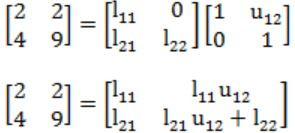l11 = 2 -----(1)
l11u12 = 2
u12 = 2/2
u12 = 1 ----- (2)
l21 = 4 ----- (3)
l21u12+l22 = 9
l22 = 9 - l21u12 = 9 - 4 × 1 = 5
 Question 16

Consider the following 2 × 2 matrix A where two elements are unknown and are marked by a and b. The eigenvalues of this matrix are –1 and 7. What are the values of a and b?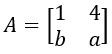A a=6, b=4 B a=4, b=6 C a=3, b=5 D a=5, b=3
Engineering-Mathematics       Linear-Algebra       GATE 2015 [Set-1]
Question 16 Explanation:
Given λ1=-1 and λ2=7 are eigen values of A
By properties,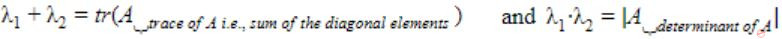⇒ 6=1+a and -7=a-4b
⇒ a=5 ⇒ -7=5-4b
⇒ b=3
 Question 17

The larger of the two eigenvalues of the matrixis _________.

 A 6 B 7 C 8 D 9
Engineering-Mathematics       Linear-Algebra       GATE 2015 [Set-2]
Question 17 Explanation:
Characteristic equation is⇒ λ2 - 5λ - 6 = 0 ⇒ (λ - 6)(λ + 1) = 0 ⇒ λ = 6, -1
∴ Larger eigen value is 6.
 Question 18

Perform the following operations on the matrix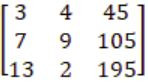(i) add the third row to the second row
(ii) Subtract the third column from the first column

The determinant of the resultant matrix is ________.

 A 0 B 1 C 2 D 3
Engineering-Mathematics       Linear-Algebra       GATE 2015 [Set-2]
Question 18 Explanation:Method 2: Determinant is unaltered by the operations (i) and (ii)
∴ Determinant of the resultant matrix = Determinant of the given matrix(Since C1, C3 are proportional i.e., C3 = 15C1)
 Question 19

In the given matrix, one of the eigenvalues is 1. the eigenvectors corresponding to the eigenvalue 1 are

```⎡ 1 -1  2 ⎤
⎢ 0  1  0 ⎥
⎣ 1  2  1 ⎦```
 A {α(4,2,1) | α≠0, α∈R} B {α(-4,2,1) | α≠0, α∈R} C {α(2,0,1) | α≠0, α∈R} D {α(-2,0,1) | α≠0, α∈R}
Engineering-Mathematics       Linear-Algebra       GATE 2015 [Set-3]
Question 19 Explanation:
X be an eigen vector corresponding to eigen value λ =1, then
AX = λX ⇒ (A - I)X = 0⇒ -y+2z = 0 and x+2y = 0
⇒ y = 2z and x/(-2) = y
∴ x/(-2) = y = 2z ⇒ x/(-4) = y/2 = z/1 = α(say)∴ Eigen vectors are {α(-4,2,1 | α≠0, α∈R}
 Question 20

If the following system has non-trivial solution,

px + qy + rz = 0
qx + ry + pz = 0
rx + py + qz = 0

then which one of the following options is True?

 A p-q+r = 0 or p = q = -r B p+q-r = 0 or p = -q = r C p+q+r = 0 or p = q = r D p-q+r = 0 or p = -q = -r
Engineering-Mathematics       Linear-Algebra       GATE 2015 [Set-3]
Question 20 Explanation:
For non-trivial solution the value of determinant should be zero.Question 21

The value of the dot product of the eigenvectors corresponding to any pair of different eigenvalues of a 4-by-4 symmetric positive definite matrix is ________.

 A 0 B 1 C 2 D 3
Engineering-Mathematics       Linear-Algebra       GATE 2014 [Set-1]
Question 21 Explanation:
For real symmetric matrix, the eigen values are orthogonal to each other. So their dot product will be zero.
The finite dimensional spectral theorem says that any symmetric matrix whose entries are real can be diagonalized by an orthogonal matrix.
 Question 22

If the matrix A is such thatthen the determinant of A is equal to

 A 0 B 1 C 2 D 3
Engineering-Mathematics       Linear-Algebra       GATE 2014 [Set-2]
Question 22 Explanation:Question 23

The product of the non-zero eigenvalues of the matrix

```1 0 0 0 1
0 1 1 1 0
0 1 1 1 0
0 1 1 1 0
1 0 0 0 1```

is ______.

 A 6 B 7 C 8 D 9
Engineering-Mathematics       Linear-Algebra       GATE 2014 [Set-2]
Question 23 Explanation:
Characteristic equation for matrix ‘A’ is
AX = λXx1 + x5 = λx1 ---------- (1)
x1 + x5 = λx5 ---------- (2)
(1) + (2) ⇒ 2(x1 + x5) = λ(x1 + x5) ⇒ λ1 = 2
x2 + x3 + x4 = λ∙x2 -------- (4)
x2 + x3 + x4 = λ∙x3 -------- (5)
x2 + x3 + x4 = λ∙x4 -------- (6)
(4)+(5)+(6) = 3(x2 + x3 + x4) = λ(x2 + x3 + x4 ) ⇒ λ2 = 3
Product = λ1 × λ2 = 2×3 = 6
 Question 24

Which one of the following statements is TRUE about every n × n matrix with only real eigenvalues?

 A If the trace of the matrix is positive and the determinant of the matrix is negative, at least one of its eigenvalues is negative. B If the trace of the matrix is positive, all its eigenvalues are positive. C If the determinant of the matrix is positive, all its eigenvalues are positive. D If the product of the trace and determinant of the matrix is positive, all its eigenvalues are positive.
Engineering-Mathematics       Linear-Algebra       GATE 2014 [Set-3]
Question 24 Explanation:
The sum of the n eigenvalues of A is the same as the trace of A (that is, the sum of the diagonal elements of A).
• The product of the n eigenvalues of A is the same as the determinant of A. •
A: Yes, for sum to be negative there should be atleast one negative number.
B: There can be one small negative number and remaining positive, where sum is positive.
C: Product of two negative numbers is positive. So, there no need of all positive eigen values.
D: There is no need for all eigen values to be positive, as product of two negative numbers is positive.
 Question 25

Which one of the following does NOT equal toABCD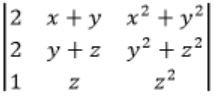Engineering-Mathematics       Linear-Algebra       GATE 2013
Question 25 Explanation:Try to derive options from the given matrix.
Observe that col 2 + col 3 will reuse x(x+1) term
C2 → C1 + C2Question 26

Let A be the 2×2 matrix with elements a11 = a12 = a21 = +1 and a22 = -1. Then the eigenvalues of the matrix A19 are

 A 1024 and -1024 B 1024√2 and -1024√2 C 4√2 and -4√2 D 512√2 and -512√2
Engineering-Mathematics       Linear-Algebra       GATE 2012
Question 26 Explanation:
a11 = a12 = a21 = 1, a22 = -1
The 2×2 matrix =Cayley Hamilton theorem:
If matrix A has ‘λ’ as eigen value, An has eigen value as λn.
Eigen value of|A-λI| = 0-(1-λ)(1+λ)-1 = 0
-(1-λ2 )-1 = 0
-1 = 1-λ2
λ2 = 2
λ = ±√2
A19 has (√2)19 = 29×√2 (or) (-√2)19 = -512√2
= 512√2
 Question 27

Consider the matrix as given below.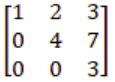Which one of the following provides the CORRECT values of eigenvalues of the matrix?

 A 1, 4, 3 B 3, 7, 3 C 7, 3, 2 D 1, 2, 3
Engineering-Mathematics       Linear-Algebra       GATE 2011
Question 27 Explanation:
Given matrix is upper triangular matrix and its diagonal elements are its eigen values = 1, 4, 3
 Question 28

Consider the following matrix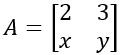. If the eigenvalues of A are 4 and 8, then

 A x=4, y=10 B x=5, y=8 C x=-3, y=9 D x=-4, y=10
Engineering-Mathematics       Linear-Algebra       GATE 2010
Question 28 Explanation:Trace = {Sum of diagonal elements of matrix}Here given that eigen values are 4, 8
Sum = 8 + 4 = 12
Trace = 2 + y
⇒ 2 + y = 12
y = 10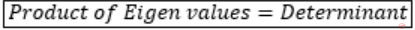Determinant = |2y - 3x|
Product of eigen values = 8 × 4 = 32
2y - 3x = 32
(y = 10)
20 - 3x = 32
-12 = 3x
x = -4
∴ x = -4, y = 10
 Question 29

The following system of equations

x1 + x2 + 2x3  = 1
x1 + 2x2 + 3x3 = 2
x1 + 4x2 + ax3 = 4

has a unique solution. The only possible value(s) for a is/are

 A 0 B either 0 or 1 C one of 0, 1 or -1 D any real number
Engineering-Mathematics       Linear-Algebra       GATE 2008
Question 29 Explanation:
The conjugate matrix [A|B] isWhen a-5 = 0, then rank(A) = rank[A|B]<3,
So infinite number of solutions.
But, it is given that the given system has unique solution i.e., rank(A) = rank[A|B] = 3 will be retain only if a-5 ≠ 0.
 Question 30

How many of the following matrices have an eigenvalue 1?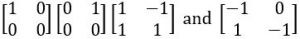A one B two C three D four
Engineering-Mathematics       Linear-Algebra       GATE 2008
Question 30 Explanation: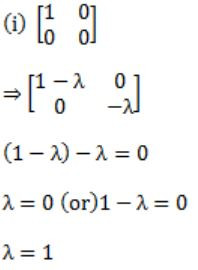Answer: We have only one matrix with eigen value 1.
 Question 31

Let A be a 4 x 4 matrix with eigenvalues -5, -2, 1, 4. Which of the following is an eigenvalue of

```[A  I]
[I  A]```

where I is the 4 x 4 identity matrix?

 A -5 B -7 C 2 D 1
Engineering-Mathematics       Linear-Algebra       GATE 2007
Question 31 Explanation:
Eigen value of A[4×4] matrix is -5, -2, 1, 4.|(A-λI)2-I| = 0 [a2-b2 = (a+b)(a-b)]
|(A-λI+I)(A-λI-I) = 0
|(A-(λ-I)I)(A-(λ+I)I| = 0
Let us assume:
λ-1=k & λ +1=k
λ =k+1 λ =k-1
⇓ ⇓
for k=-5; λ=-4 λ =-6
k=-2; λ=-1 λ =-3
k=1; λ=2 λ = 0
k=4; λ=5 λ = 3
So, λ = -4,-1,2,5,-6,-3,0,3
Check with the option
Option C = 2
 Question 32

Consider the set of (column) vectors defined by X = {x ∈ R3| x1+x2+x3 = 0, where xT = [x1,x2,x3]T}. Which of the following is TRUE?

 A {[1,-1,0]T, [1,0,-1]T} is a basis for the subspace X. B {[1,-1,0]T, [1,0,-1]T} is a linearly independent set, but it does not span X and therefore is not a basis of X. C X is not a subspace of R3 D None of the above
Engineering-Mathematics       Linear-Algebra       GATE 2007
Question 32 Explanation:
Since, [1,-1,0]T and [1,0,-1]T are linearly independent set and spans X. So is the basis for subspace X.
 Question 33

F is an n×n real matrix. b is an n×1 real vector. Suppose there are two n×1 vectors, u and v such that, u≠v and Fu=b, Fv=b. Which one of the following statements is false?

 A Determinant of F is zero B There are an infinite number of solutions to Fx=b C There is an x≠0 such that Fx=0 D F must have two identical rows
Engineering-Mathematics       Linear-Algebra       GATE 2006
Question 33 Explanation:
⇾ Fu = b, Fv = b
Fu = Fv
Fu - Fv = 0
F(u - v) = 0
Given u ≠ v
F = 0 (i.e., Singular matrix, so determinant is zero)
Option A is true.
⇾ Fx = b; where F is singular
It can have no solution (or) infinitely many solutions.
Option B is true.
⇾ x ≠ 0 such that Fx = 0 is True because F is singular matrix (“stated by singular matrix rules). Option C is true.
⇾ F can two identical columns and rows.
Option D is false.
 Question 34

Consider the following system of equations in three real variables xl, x2 and x3

```2xl - x2 + 3x3 = 1
3xl- 2x2 + 5x3 = 2
-xl + 4x2 + x3 = 3 ```

This system of equations has

 A no solution B a unique solution C more than one but a finite number of solutions D an infinite number of solutions
Engineering-Mathematics       Linear-Algebra       GATE 2005
Question 34 Explanation:2(-2 - 20) +1(3 + 5) + 3(12 - 2)
= -44 + 8 + 30
= -6 ≠ 0
→ |A| ≠ 0, we have Unique Solution.
 Question 35

What are the eigenvalues of the following 2 × 2 matrix?

```|2   -1|
|-4   5| ```
 A -1 and 1 B 1 and 6 C 2 and 5 D 4 and -1
Engineering-Mathematics       Linear-Algebra       GATE 2005
Question 35 Explanation: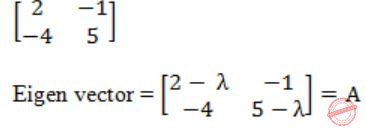|A| = (2 - λ)(5 - λ) - (4) = 0
10 - 7λ+ λ2 - 4 = 0
λ2 - 7λ + 6 = 0
λ2 - 6λ - λ + 6 = 0
(λ - 6) -1(λ - 6) = 0
λ = 1 (or) 6
 Question 36

The number of different n × n symmetric matrices with each element being either 0 or 1 is: (Note: power(2,x) is same as 2x)

 A power (2,n) B power (2,n2) C power (2, (n2 + n)/2) D power (2, (n2 - n)/2)
Engineering-Mathematics       Linear-Algebra       GATE 2004
Question 36 Explanation:
If a matrix is symmetric then
A[i][j] = A[j][i]
So, we have only two choices, they are either upper triangular elements (or) lower triangular elements.
No. of such elements are
n + (n-1) + (n-2) + ... + 1
n(n+1)/2
We have two choices, thus we have
2(n(n+1)/2) = 2((n2+n)/2) choices
i.e., Power (2, (n2+n)/2).
 Question 37

Let A, B, C, D be n × n matrices, each with non-­zero determinant. If ABCD = 1, then B-1 is

 A D-1C-1A-1 B CDA C ADC D Does not necessarily exist
Engineering-Mathematics       Linear-Algebra       GATE 2004
Question 37 Explanation:
ABCD are n × n matrices with non-zero determinant.
ABCD = I
Pre multiply A-1 on both sides
A-1ABCD = A-1⋅I
BCD = A-1
Pre multiply B-1 on both sides
B-1BCD = B-1A-1
CD = B-1A-1
Post multiply A on both sides
CDA = B-1A-1⋅A
∴ CDA = B-1(I)
∴ CDA = B-1
 Question 38

How many solutions does the following system of linear equations have?

```  -x + 5y = -1
x - y = 2
x + 3y = 3 ```
 A infinitely many B two distinct solutions C unique D none
Engineering-Mathematics       Linear-Algebra       GATE 2004
Question 38 Explanation:rank = r(A) = r(A|B) = 2
rank = total no. of variables
Hence, unique solution.
There are 38 questions to complete.

Register Now